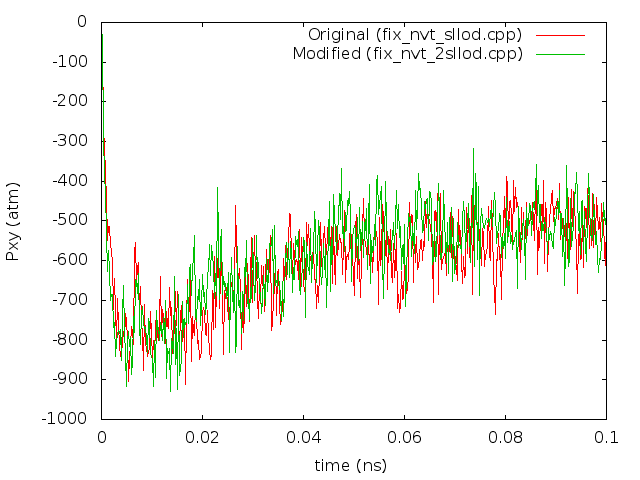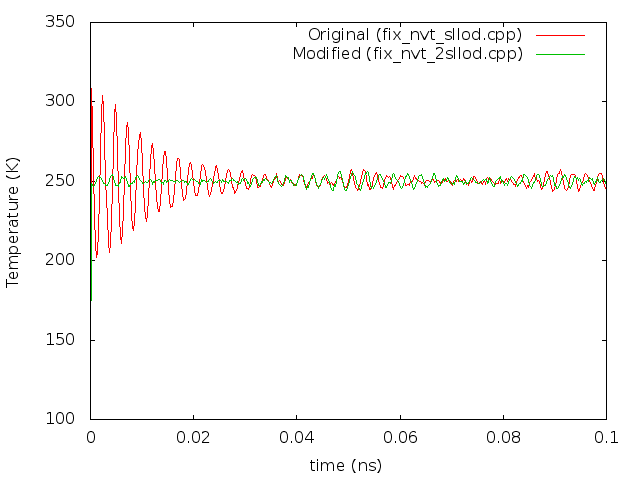# Some observations about SLLOD

Hello Users and Developers,

I have recently been looking into the SLLOD implementation with interest in making a new “fix” which will integrate the SLLOD equations in the NPT ensemble (of type “iso”) and I noticed some details about the current implementation which I though were worth mentioning. I think there might be a small inconsistency in the SLLOD code as well, so if you are interested only in that, please skip to 2.

1. The current version of SLLOD is thermostat driven, which causes oscillations during startup.
I’m pretty sure you already know that this is the case, but I’ll explain anyway. If SLLOD is turned on without the presence of an initial flow field, the velocities will be thermostatted to the streaming velocities. This will result in some temperature oscillations due to the thermostat until the streaming velocities are obtained. One way to avoid this would be to impose an initial velocity profile over the thermal velocities which were present before the fix_deform command began.

Another approach is to remap positions when the box is deformed, and integrate the original velocities as thermal velocities. I implemented this approach in the files “fix_nvt_2sllod(.h/.cpp)” (which requires a fix_deform command with the option “remap x”, no need to remap v because the velocities are thermal. This also allows for use of unbiased calculation of the temperature and pressure in the system, but this is only a minor convenience. I made a plot for a comparison of the temperatures for the two methods during startup of shear for a polymer system in “T_shear.png” to show the oscillations in the original method, and also a plot of Pxy in “Pxy_shear.png” which seems to show the same behaviour for both methods. I did notice that my version ran a little faster, around ~3% (maybe there is some overhead associated with remapping velocities?), but this may be due to the oscillations in the original implementation which will become less important with time. Maybe this form of the fix is something to look into?

1. I think the current version of SLLOD is actually GSLLOD (which is the same for shear, but not in general)
I think this may have been inadvertent, based on the following code and comments on “fix_nvt_sllod.cpp”:

comment on line 103:
// vdelu = SLLOD correction = HrateH_invvthermal

code to implement this lines 115-129:

double h_two,vdelu;
MathExtra::multiply_shape_shape(domain->h_rate,domain->h_inv,h_two);

for (int i = 0; i < nlocal; i++) {
if (mask[i] & groupbit) {
vdelu = h_two*v[i] + h_two*v[i] + h_two*v[i];
vdelu = h_two*v[i] + h_two*v[i];
vdelu = h_two*v[i];
temperature->remove_bias(i,v[i]);
v[i] = v[i]factor_eta - dthalfvdelu;
v[i] = v[i]factor_eta - dthalfvdelu;
v[i] = v[i]factor_eta - dthalfvdelu;
temperature->restore_bias(i,v[i]);
}
}

Notice that vdelu is calculated before the bias is removed, but the comment mentions that the correction should be using vthermal. I believe this makes the current scheme equivalent to GSLLOD because the velocity contains the streaming velocity (q.delu) and the velocity relative to it (vthermal):
v = q.delu + vthermal
When this is multiplied by delu, this means that as calculated, vdelu above is equal to:
q.(delu).(delu) + vthermal(delu)
which is the correction for the GSLLOD equations of motion. To get the regular SLLOD equations, I think the “temperature->remove_bias(i,v[i]);” line could just be moved up above the calculation of vdelu (I tried this fix, but only have done shear simulations which resulted in the same behaviour, as expected, until divergence due to numerics took over after about 20 ps).

P.S. I also have a preliminary version of SLLOD with NPT integration if anyone is interested; just let me know.

Thanks,
Davidfix_nvt_2sllod.cpp (3.34 KB)

fix_nvt_2sllod.h (1.1 KB)

Steve

Hello Users and Developers,

I have recently been looking into the SLLOD implementation with interest in
making a new "fix" which will integrate the SLLOD equations in the NPT
ensemble (of type "iso") and I noticed some details about the current
implementation which I though were worth mentioning. I think there might be
a small inconsistency in the SLLOD code as well, so if you are interested

1) The current version of SLLOD is thermostat driven, which causes
oscillations during startup.
I'm pretty sure you already know that this is the case, but I'll explain
anyway. If SLLOD is turned on without the presence of an initial flow field,
the velocities will be thermostatted to the streaming velocities. This will
result in some temperature oscillations due to the thermostat until the
streaming velocities are obtained. One way to avoid this would be to impose
an initial velocity profile over the thermal velocities which were present
before the fix_deform command began.

The velocity ramp command can be used to do that.

Another approach is to remap positions when the box is deformed, and
integrate the original velocities as thermal velocities. I implemented this
approach in the files "fix_nvt_2sllod(.h/.cpp)" (which requires a fix_deform
command with the option "remap x", no need to remap v because the velocities
are thermal. This also allows for use of unbiased calculation of the
temperature and pressure in the system, but this is only a minor
convenience. I made a plot for a comparison of the temperatures for the two
methods during startup of shear for a polymer system in "T_shear.png" to
show the oscillations in the original method, and also a plot of Pxy in
"Pxy_shear.png" which seems to show the same behaviour for both methods. I
did notice that my version ran a little faster, around ~3% (maybe there is
some overhead associated with remapping velocities?), but this may be due to
the oscillations in the original implementation which will become less
important with time. Maybe this form of the fix is something to look into?

That's an interesting idea. Conceptually we thought of "remap x" for solids
and "remap v" for liquids, which is where you would use SLLOD. I'd have
to convince myself there are no diagnostic or other issues that rely on the
liquid case actually having atoms with a streaming velocity. Pxy for
example does not
rely on the differential v's in the kinetic component? Shearing a granular
system does I believe, b/c the granular forces have a relative velocity
component, which remap x would be removing.

2) I think the current version of SLLOD is actually GSLLOD (which is the
same for shear, but not in general)
I think this may have been inadvertent, based on the following code and

comment on line 103:
// vdelu = SLLOD correction = Hrate*H_inv*vthermal

code to implement this lines 115-129:
double h_two,vdelu;
MathExtra::multiply_shape_shape(domain->h_rate,domain->h_inv,h_two);

for (int i = 0; i < nlocal; i++) {
if (mask[i] & groupbit) {
vdelu = h_two*v[i] + h_two*v[i] + h_two*v[i];
vdelu = h_two*v[i] + h_two*v[i];
vdelu = h_two*v[i];
temperature->remove_bias(i,v[i]);
v[i] = v[i]*factor_eta - dthalf*vdelu;
v[i] = v[i]*factor_eta - dthalf*vdelu;
v[i] = v[i]*factor_eta - dthalf*vdelu;
temperature->restore_bias(i,v[i]);
}
}

Notice that vdelu is calculated before the bias is removed, but the comment
mentions that the correction should be using vthermal. I believe this makes
the current scheme equivalent to GSLLOD because the velocity contains the
streaming velocity (q.delu) and the velocity relative to it (vthermal):
v = q.delu + vthermal
When this is multiplied by delu, this means that as calculated, vdelu above
is equal to:
q.(delu).(delu) + vthermal(delu)
which is the correction for the GSLLOD equations of motion. To get the
regular SLLOD equations, I think the "temperature->remove_bias(i,v[i]);"
line could just be moved up above the calculation of vdelu (I tried this
fix, but only have done shear simulations which resulted in the same
behaviour, as expected, until divergence due to numerics took over after